### Simulation of Queuing Systems

Simulation of Queuing systems

• The calling population, the nature of the arrivals, the service mechanism, the system capacity, and the queueing discipline all contribute to the description of a queueing system.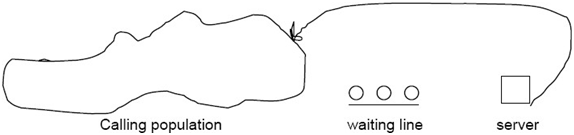Figure 1: Queueing System

• The calling population in a single-channel queue is limitless; that is, if a unit leaves the calling population and joins the waiting line or enters service, the arrival rate of subsequent units that may need service does not change.

• Arrivals for service happen one at a time, at random intervals; once they join the waiting line, they will be served eventually. Furthermore, service periods are of a random length determined by a probability distribution that remains constant throughout time.

• The system capacity is unlimited, which means that any number of units can queue.

• Finally, a single server or channel serves units in the order in which they arrive.

• The distributions of time between arrivals and the distribution of service times, respectively, characterize arrivals and services.

• The entire effective arrival rate must be less than the total service rate for any simple single or multi-channel queue, else the waiting line will extend indefinitely. The term "explosive" or "unstable" refers to queues that expand without limit.

• The number of units in the system and the condition of the server (busy or idle) make up the system state.

• There are just two conceivable events that can influence the state of a single-channel queueing system.

1. They are the entry of a unit into the system.

2. The completion of service on a unit.

• The queueing system includes the server, the unit being serviced, and units in the queue. He simulation clock is used to track simulated time.

•  If a unit has just completed service, the simulation proceeds in the manner shown in the flow diagram of figure.2. Note that the server has only two possible states: it is either busy or idle.

​​​​​​​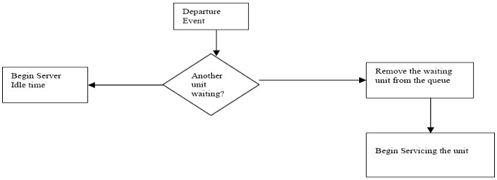Figure 2: Service-just-completed flow diagram

• The arrival event occurs when a unit enters the system. The flow diagram for the arrival event is shown in figure 3. The unit may find the server either idle or busy; therefore, either the unit begins service immediately, or it enters the queue for the server.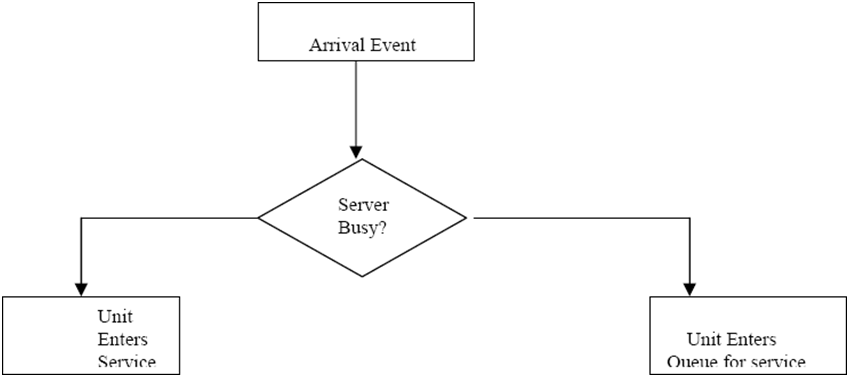Figure 3: Unit-Entering system flow diagram

• If the server is overloaded, the unit joins the queue. The unit starts serving if the server is idle and the queue is empty. It's impossible for the server to be idle while the queue isn't full.

​​​​​​​​​​​​​​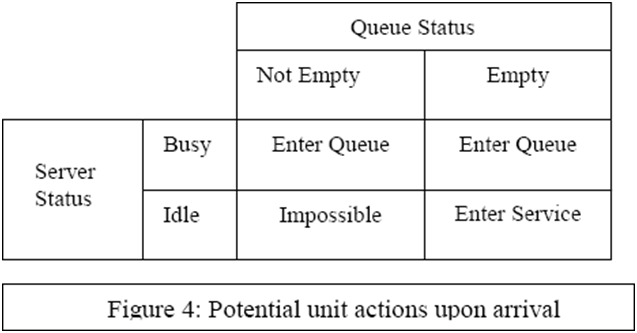• The service may stay idle when a service is completed, or it may remain busy with the following unit. Figure 5 depicts the relationship between these two outcomes and the queue's state. If the queue is not empty, another unit will join it, making the server busy. After a service is performed, the server will be idle if the queue is empty. The darkened areas of fig 5 depict these two possibilities. When a service is completed, the server cannot get busy since the queue is empty. When the queue is not empty, it is also difficult for the server to be idle once a service is performed.

​​​​​​​​​​​​​​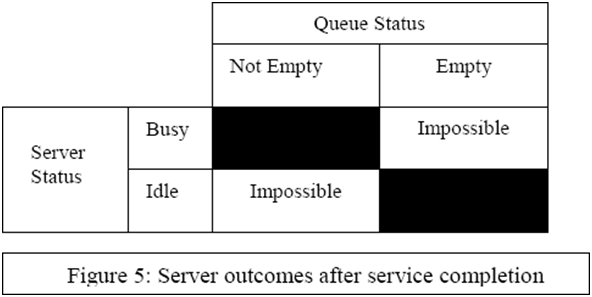• Arrival and departure simulation clock periods are calculated in a simulation table that is tailored for each scenario. Events in simulations frequently happen at random times. In these situations, a statistical model of the data is created using either acquired and processed data or subjective estimations and assumptions.

• On the interval, random numbers are dispersed uniformly and independently (0, 1). The digits 0 through 9 are evenly dispersed across the set. By picking the appropriate amount of digits for each random number and inserting a decimal point to the left of the value selected, random digits can be used to generate random numbers. The number of digits required is determined on the accuracy of the data being input. If the input distribution includes two decimal places, two digits from a random-digits table are picked and the decimal point is moved to the left to generate a random number.

• Pseudorandom numbers are generated using a process and are typically referred to as such. Because the process is known, the sequence of numbers that will be generated prior to the simulation can always be predicted.

• Interarrival times and service times are calculated using the distributions of these random variables in a single-channel queueing system. The following examples demonstrate how such times are generated. Assume that the times between arrivals were created by rolling a die five times and recording the up face for simplicity. Table 1 shows five interarrival times that were utilized to calculate the arrival times of six consumers at the queue system.

Table 1: Interarrival and Clock Times